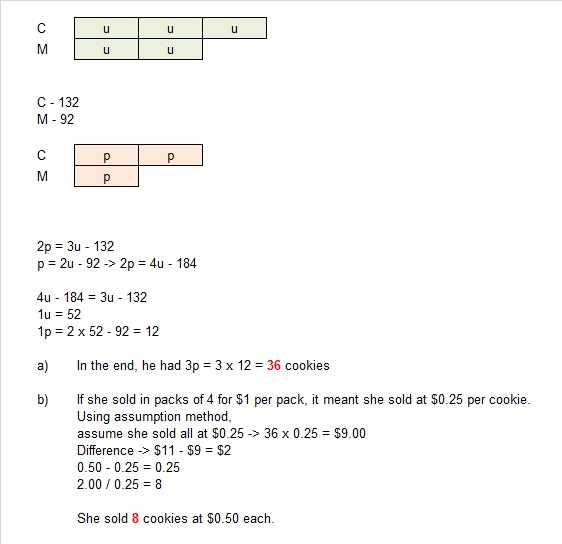# Question

(a) How many cookies did she have altogether in the end?

(b) The remaining cookies were sold in packets of 4 for \$1 or \$0.50 each. She earned \$11 from the sales. How many cookies were sold at \$0.50 each?

This is a Before After question.

We shall first tabulate the information.

 Chocolate Macadamia Total Before 3u 2u 5u After 3u – 132 2u – 92 5u – 224

The last ratio is 2: 1.

(3u -132) / 2  = (2u – 92) / 1

3u – 132 = 4u – 184

184 – 132 = 4u – 3u

1u = 52

(a)   5u = 5 × 52 = 260

260 – 224 = 36

(b)

(1u x 0.5) + (36 – 1u) × 0.25 =  11

0.5u + 9 – 0.25u = 11

0.25u = 2

1u = 2 ÷ 0.25 = 8

0 Replies 1 Like